Data Types  «Prev  Next»
 Lesson 4 Convert Decimal to Binary Objective Convert numbers between decimal and binary

# Converting Decimal to Binary

Converting a decimal number to its binary equivalent is a little more challenging. Here's one approach.
1. Find the highest power of 2 less than or equal to the decimal number. In your binary number, place a 1 in the position corresponding to this power of 2.
2. Subtract the power of 2 found in step 1 from the decimal number to obtain a new decimal number. If the new decimal number is 0, then you are done; otherwise, return to step 1 with the new decimal number.

Click through the following SlideShow to convert the decimal number 204 to binary.

## Converting from decimal to binary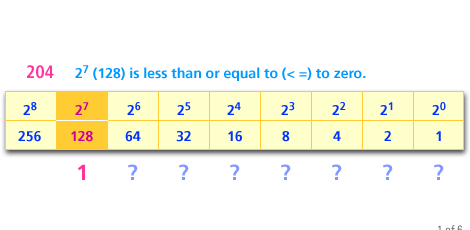1) The largest power of 2 less than or equal to 204 is 2 raised to the 7th = 128. Thus the binary number should have a 1 in the position corresponding to 2^7.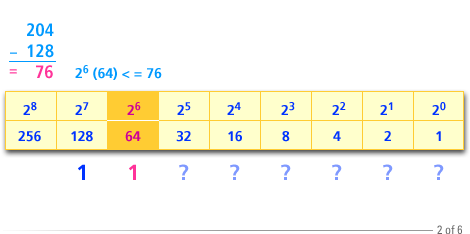2) Subtracting 128 from 204 gives 76. The largest power of 2 less than or equal to 76 is 2 raised to the 6th = 64. Thus the binary number should have a 1 in the position corresponding to 2^6.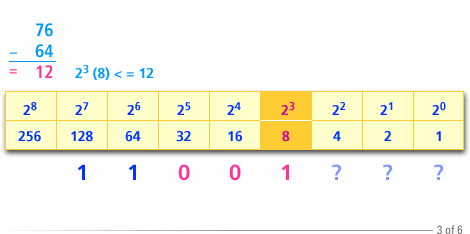3) Subtracting 64 from 76 gives 12. The largest power of 2 less than or equal to 12 is 2 raised to the 3 = 8. Thus the binary number should have a 1 in the position corresponding to 2^3.
Computer Science Structured Approach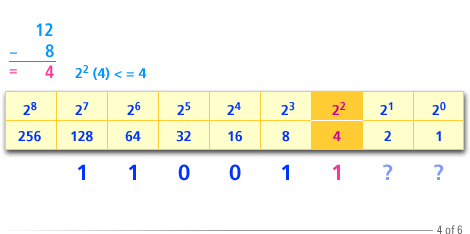4) Subtracting 8 from 12 gives 4. The largest power of 2 less than or equal to 4 is 2^2 =4. Thus the binary should have a 1 in the position corresponding to 2^2.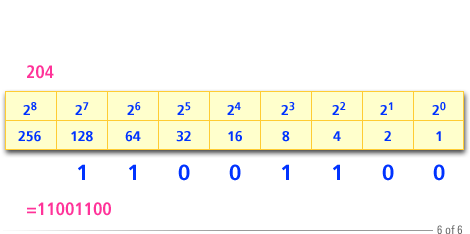6) From our work we see that the binary equivalent of 204 should have 1s in the corresponding to 2^7, 2^6, 2^3 and 2^2. All other positions should contain 0's. Thus the binary equivalent of 204 is 11001100.

1. The largest power of 2 less than or equal to 204 is 2 raised to the 7th = 128.
2. Subtracting 128 from 204 gives 76. The largest power of 2 less than or equal to 76 is 2 raised to the 6th = 64.
3. Subtracting 64 from 76 gives 12. The largest power of 2 less than or equal to 12 is 2 raised to the 3 = 8.
4. Subtracting 8 from 12 gives 4. The largest power of 2 less than or equal to 4 is 2^2 =4.
5. Subtracting 4 from 4 givess 0. We are completed.
6. From our work we see that the binary equivalent of 204 should have 1s in the corresponding to 2^7, 2^6, 2^3 and 2^2.

Binary numbers can require many digits. In the next lesson, you will look at how binary numbers can be expressed more compactly using hexadecimal form.

### Converting Binary Numbers - Quiz

Click the Quiz link below to see how well you understand binary numbers.
Converting Binary Numbers - Quiz